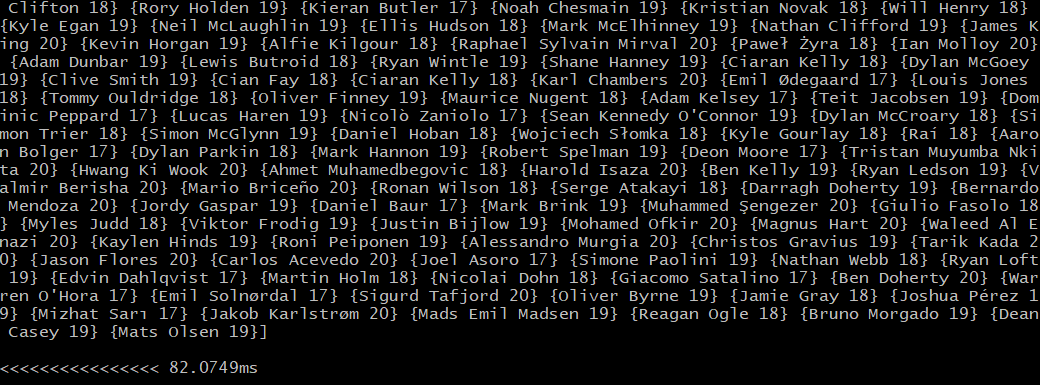## 1. 用例

``````// 示例PlayerInfo结构
type PlayerInfo struct {
// ID          string  `json:"ID"`
// PlayerID    string  `json:"PlayerID"`
Name        string  `json:"Name"`
Age         int     `json:"Age"`
}
``````

## 2. 开启档案

``````// 打开文件
f, err := os.Open(absPath)
if err != nil {
panic(err)
}
defer f.Close()

if err != nil {
panic(err)
}
``````

## 3. 建立频道

``````// 实施渠道
lists := make(chan []PlayerInfo)

finalValue := make(chan []PlayerInfo)

var wg sync.WaitGroup
``````

## 4. 映射器实现

``````// Map -
func Map(player []string) []PlayerInfo {
list := []PlayerInfo{}
age, _ := strconv.Atoi(player)
list = append(list, PlayerInfo{
// ID:   player,
Name: player,
Age:  age,
})
return list
}
``````

## 5. 减速器实施

``````// Reducer -
func Reducer(mapList chan []PlayerInfo, sendFianlValue chan []PlayerInfo) {
final := []PlayerInfo{}
for list := range mapList {
for _, value := range list {
if value.Age <= 20 {
final = append(final, value)
}
}
}
sendFianlValue <- final
}
``````

## 6. 使用goroutine调用mapper和reducer

``````var wg sync.WaitGroup
beginTime := time.Now()
for _, line := range lines {
go func(player []string) {
defer wg.Done()
lists <- Map(player)
}(line)
}

go Reducer(lists, finalValue)
wg.Wait()
close(lists)

fmt.Println(<-finalValue)
fmt.Println("\n<<<<<<<<<<<<<<<<", time.Now().Sub(beginTime))
``````

## 7. 结果